# How to round numbers in Excel - ROUND, ROUNDUP, ROUNDDOWN and other functions

The tutorial explains the uses of ROUND, ROUNDUP, ROUNDDOWN, FLOOR, CEILING, MROUND and other Excel rounding functions and provides formula examples to round decimal numbers to integers or to a certain number of decimal places, extract a fractional part, round to nearest 5, 10 or 100, and more.

In some situations when you don't need an exact answer, rounding is a useful skill to use. In plain English, to round a number is to eliminate the least significant digits, making it simpler but keeping close to the original value. In other words, rounding lets you get an approximate number with the desired level of accuracy.

In everyday life, rounding is commonly used to make numbers easier to estimate, communicate or work with. For instance, you can use rounding to make long decimal numbers shorter to report the results of complex calculations or round off currency values.

Many different ways of rounding exist, such as rounding to integer, rounding to a specified increment, rounding to simple fractions, and so on. And Microsoft Excel provides a handful of functions to handle different rounding types. Below, you will find a quick overview of the major round functions and well as formula examples that demonstrate how to use those functions on the real-life data in your worksheets.

## Excel rounding by changing the cell format

If you want to round numbers in Excel solely for presentations purposes, you can change the cell's format by performing the following steps:

1. Select the cell with the number(s) you want to round.
2. Open the Format Cells dialog by pressing Ctrl + 1 or right click the cell(s) and choose Format Cells... from the context menu.
3. In the Format Cells window, switch to either Number or Currency tab, and type the number of decimal places you want to display in the Decimal paces box. A preview of the rounded number will immediately show up under Sample.
4. Click the OK button to save the changes and close the Format Cells dialog.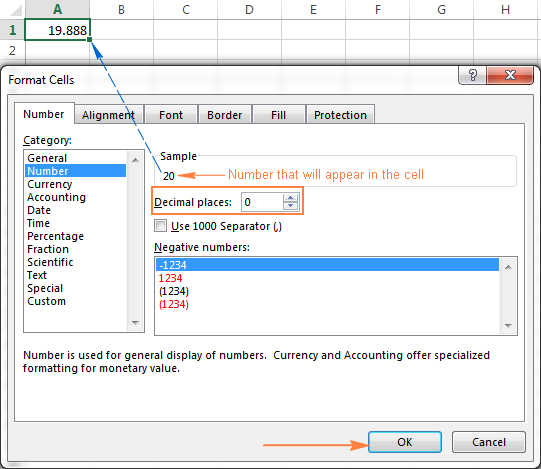Important note! This method changes the display format without changing the actual value stored in a cell. If you refer to that cell in any formulas, the original non-round value will be used in all calculations.

## Excel functions to round numbers

Unlike formatting options that change only the display value, Excel round functions alter the actual value in a cell.

Below you will find a list of functions specially designed for performing different types of rounding in Excel.

• ROUND - round the number to the specified number of digits.
• ROUNDUP - round the number upward to the specified number of digits.
• ROUNDDOWN - round the number downward to the specified number of digits.
• MROUND - rounds the number upward or downward to the specified multiple.
• FLOOR - round the number down to the specified multiple.
• CEILING - round the number up to the specified multiple.
• INT - round the number down to the nearest integer.
• TRUNC - truncate the number to a specified number of decimal places.
• EVEN - round the number up to the nearest even integer.
• ODD - round the number up to the nearest odd integer.

### Excel ROUND function

ROUND is the major rounding function in Excel that rounds a numeric value to a specified number of digits.

Syntax: ROUND(number, num_digits)

Number - any real number you want to round. This can be a number, reference to a cell containing the number or a formula-driven value.

Num_digits - the number of digits to round the number to. You can supply a positive or negative value in this argument:

• If num_digits is greater than 0, the number is rounded to the specified number of decimal places.

For example `=ROUND(15.55,1)` rounds 15.55 to 15.6.

• If num_digits is less than 0, all decimal places are removed and the number is rounded to the left of the decimal point (to the nearest ten, hundred, thousand, etc.).

For example `=ROUND(15.55,-1)` rounds 15.55 to the nearest 10 and returns 20 as the result.

• If num_digits equals 0, the number is rounded to the nearest integer (no decimal places).

For example `=ROUND(15.55,0)` rounds 15.55 to 16.

The Excel ROUND function follows the general math rules for rounding, where the number to the right of the rounding digit determines whether the number is rounded upwards or downwards.

Rounding digit is the last significant digit retained once the number is rounded, and it gets changed depending on whether the digit that follows it is greater or less than 5:

• If the digit to the right of the rounding digit is 0, 1, 2, 3, or 4, the rounding digit is not changed, and the number is said to be rounded down.
• If the rounding digit is followed by 5, 6, 7, 8, or 9, the rounding digit is increased by one, and the number is rounded up.

The following screenshot demonstrates a few ROUND formula examples: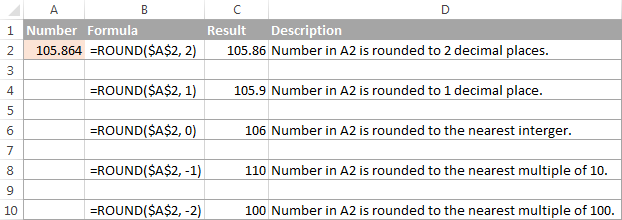### Excel ROUNDUP function

The ROUNDUP function rounds the number upward (away from 0) to a specified number of digits.

Syntax: ROUNDUP(number, num_digits)

Number - the number to be rounded up.

Num_digits - the number of digits you want to round the number to. You can supply both positive and negative numbers in this argument, and it works like num_digits of the ROUND function discussed above, except that a number is always rounded upward.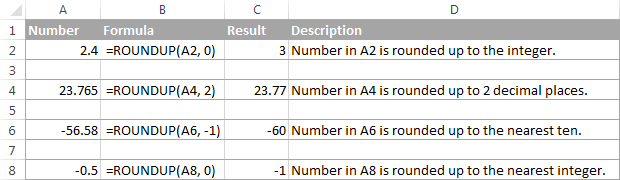### Excel ROUNDDOWN function

The ROUNDDOWN function in Excel does the opposite of what ROUNDUP does, i.e. rounds a number down, toward zero.

Syntax: ROUNDDOWN(number, num_digits)

Number - the number to be rounded down.

Num_digits - the number of digits you want to round the number to. It works like the num_digits argument of the ROUND function, except that a number is always rounded downward.

The following screenshot demonstrates the ROUNDDOWN function in action.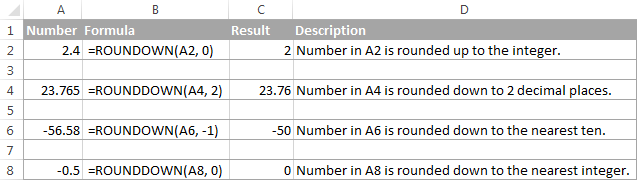### Excel MROUND function

The MROUND function in Excel rounds a given number up or down to the specified multiple.

Syntax: MROUND(number, multiple)

Number - the value you want to round.

Multiple - the multiple to which you want to round the number.

For example, the formula `=MROUND(7, 2)` rounds 7 to the nearest multiple of 2 and returns 8 as the result.

Whether the last remaining digit is rounded up (away from 0) or down (towards 0) depends on the remainder from dividing the number argument by the multiple argument:

• If the remainder is equal to or greater than half the value of the multiple argument, Excel MROUND rounds the last digit up.
• If the remainder is less than half the value of the multiple argument, the last digit is rounded down.

The MROUND function comes in handy, say, for rounding prices to the nearest nickel (5 cents) or a dime (10 cents) to avoid dealing with pennies as change.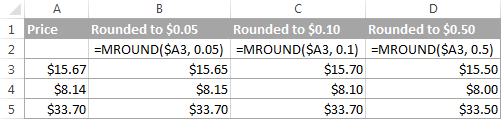And, it is really indispensable when it comes to rounding times to a desired interval. For example, to round time to the nearest 5 or 10 minutes, just supply "0:05" or "0:10" for the multiple, like this:

`=MROUND(A2,"0:05")` or `=MROUND(A2,"0:10")`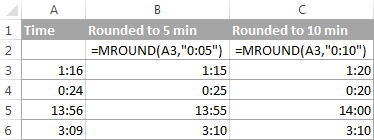Note. The MROUND function returns the #NUM! error when its arguments have different signs. For example, both of the formulas `=MROUND(3, -2)` and `=MROUND(-5, 2)` result in the NUM error.

### Excel FLOOR function

The FLOOR function in Excel is used to round a given number down, to the nearest multiple of a specified significance.

Syntax: FLOOR(number, significance)

Number - the number you want to round.

Significance - the multiple to which you wish to round the number.

For example, `=FLOOR(2.5, 2)` rounds 2.5 down to the nearest multiple of 2, which is 2.

The Excel FLOOR function performs rounding based on the following rules:

• If the number and significance arguments are positive, the number is rounded down, toward zero, as in rows 2 and 10 in the screenshot below.
• If number is positive and significance is negative, the FLOOR function returns the #NUM error, as in row 4.
• If number is negative and significance is positive, the value is rounded down, away from zero, as in row 6.
• If number and significance are negative, the number is rounded up, toward zero, as in row 8.
• If number is an exact multiple of the significance argument, no rounding takes place.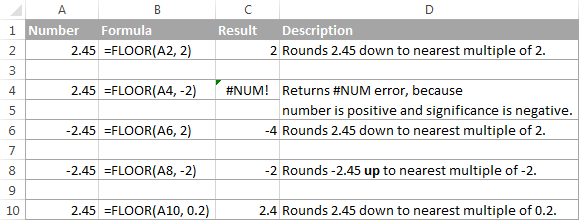Note. In Excel 2003 & 2007, the number and significance arguments must have the same sign, either positive or negative, otherwise an error is returned. In newer Excel versions, the FLOOR function has been improved, so in Excel 2010, 2013 and 2016 it can handle a negative number and positive significance.

### Excel CEILING function

The CEILING function in Excel rounds a given number up, to the nearest multiple of significance. It has the same syntax as the FLOOR function.

Syntax: CEILING(number, significance)

Number - the number you want to round.

Significance - the multiple to which you want to round the number.

For instance, the formula `=CEILING(2.5, 2)` rounds 2.5 up to the nearest multiple of 2, which is 4.

The Excel CEILING function works based on the rounding rules similar to FLOOR's, except that it generally rounds up, away from 0.

• If both the number and significance arguments are positive, the number is rounded up, as in rows 2 and 10 in the screenshot below.
• If number is positive and significance is negative, the CEILING function returns the #NUM error, as in row 4.
• If number is negative and significance is positive, the value is rounded up, towards zero, as in row 6.
• If number and significance are negative, the value is rounded down, as in row 8.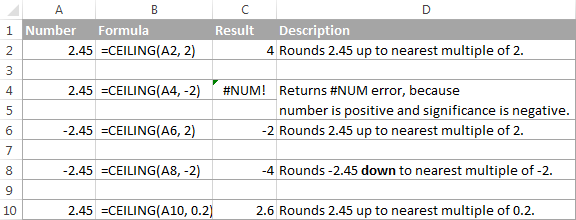### Excel INT function

The INT function rounds a number down to the nearest integer.

Of all Excel round functions, INT is probably the easiest one to use, because it requires only one argument.

Syntax: INT(number)

Number - the number you want to round down to the nearest integer.

Positive numbers are rounded toward 0 while negative numbers are rounded away from 0. For example, `=INT(1.5)` returns 1 and `=INT(-1.5)` returns -2.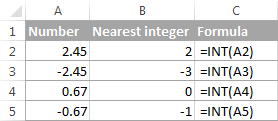### Excel TRUNC function

The TRUNC function truncates a given numeric value to a specified number of decimal places.

Syntax: TRUNC(number, [num_digits])

• Number - any real number that you want to truncate.
• Num_digits - an optional argument that defines the precision of the truncation, i.e. the number of decimal places to truncate the number to. If omitted, the number is truncated to an integer (zero decimal places).

The Excel TRUNC function adheres to the following rounding rules:

• If num_digits is positive, the number is truncated to the specified number of digits to the right of the decimal point.
• If num_digits is negative, the number is truncated to the specified number of digits to the left of the decimal point.
• If num_digits is 0 or omitted, it rounds the number to an integer. In this case, the TRUNC function works similarly to INT in that both return integers. However, TRUNC simply removes the factional part, while INT rounds a number down to the nearest integer.For example, `=TRUNC(-2.4)` returns -2, while `=INT(-2.4)` returns -3 because it's the lower integer. For more info, please see Rounding to integer example.

The following screenshot demonstrates the TRUNC function in action: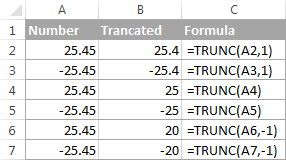### Excel ODD and EVEN functions

These are two more functions provided by Excel for rounding a specified number to an integer.

ODD(number) rounds up to the nearest odd integer.

EVEN(number) rounds up to the nearest even integer.

• In both functions, number is any real number that you want to round.
• If number is non-numeric, the functions return the #VALUE! error.
• If number is negative, it is rounded away from zero.

The ODD and EVEN functions may prove useful when you are processing items that come in pairs.

For example:

`=ODD(2.4)` returns 3

`=ODD(-2.4)` returns -3

`=EVEN(2.4)` returns 4

`=EVEN(-2.4)` returns -4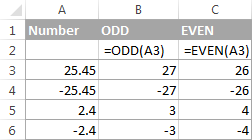## Using rounding formulas in Excel

As you see, there exist a variety of functions to round off numbers in Excel depending on the particular purpose. The following examples will hopefully give you some clues on how to use Excel rounding formulas based on your criteria.

### How to round decimals in Excel to a certain number of places

Depending on the situation, you may want to round decimals up, down or based on math rounding rules:

ROUNDUP function - always rounds the decimal upward.

ROUNDDOWN function - always rounds the decimal downward.

ROUND - rounds up if the rounding digit is followed by the digit equal to or greater than 5, otherwise rounds down.

As an example, lets round the decimal numbers in column A to 2 decimal places. In the first argument (number), you enter a reference to the cell containing the number, and in the second argument (num_digits) you specify the number of decimal places you want to keep.

`=ROUNDUP(A2, 2)` - rounds the number in A2 upward, to two decimal places.

`=ROUNDDOWN(A2, 2)` - rounds the number in A2 downward, to two decimal places.

`=ROUND(A2, 2)` - rounds the number in A2 to 2 decimal places, upward or downward, depending on whether the 3rd decimal digit is greater or less than 5.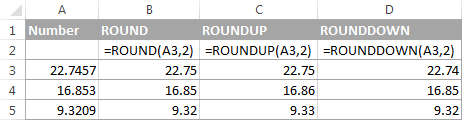### Rounding negative numbers (ROUND, ROUNDDOWN, ROUNDUP)

When it comes to rounding a negative number, the results returned by the Excel round functions, may seem to flout logic :)

When the ROUNDUP function applies to negative numbers, they are said to be rounded up, even though they actually decrease in value. For example, the result of `=ROUNDUP(-0.5, 0)` is -1, as in row 7 in the screenshot below.

The ROUNDDOWN function is known to round numbers downward, though negative numbers may increase in value. For example, the formula `=ROUNDDOWN(-0.5, 0)` returns 0, as in row 8 in the screenshot below.

In fact, the rounding logic with regard to negative numbers is very simple. Whenever you use the ROUND, ROUNDDOWN or ROUNDUP function in Excel on a negative number, that number is first converted to its absolute value (without the minus sign), then the rounding operation occurs, and then the negative sign is re-applied to the result.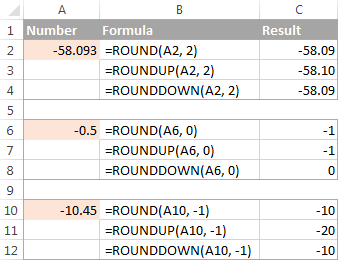### How to extract a decimal part of a number

If you want to extract a fractional part of a decimal number, you can use the TRUNC function to truncate the decimal places and then subtract that integer from the original decimal number.

`=A2 - TRUNC(A2,0)`

As demonstrated in the screenshot below, the formula in column B works perfectly both for positive and negative numbers. However, if you'd rather get an absolute value (decimal part without the minus sign), then wrap the formula in the ABS function:

`=ABS(A2 - TRUNC(A2,0))`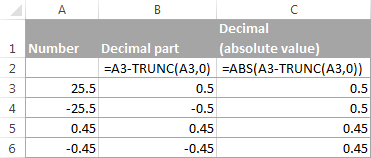### How to round a decimal to an integer in Excel

As is the case with rounding to a certain number of decimal places, there is a handful of functions for rounding a fractional number to an integer.

#### ROUNDUP

To round up to nearest integer, use an Excel ROUNDUP formula with num_digits set to 0. For example `=ROUNDUP(5.5, 0)` rounds decimal 5.5 to 6.

#### INT or ROUNDDOW

To round down to nearest whole number, use either INT or ROUNDDOW with num_digits set to 0. For example both of the following formulas round 5.5 to 5:

`=ROUNDOWN(5.5, 0)`

`=INT(5.5)`

For negative decimals, however, the INT and ROUNDDOWN functions yield different results - INT rounds negative decimals away from 0, while ROUNDDOWN toward 0:

`=ROUNDOWN(-5.5, 0)` returns -5.

`=INT(-5.5)` returns -6.

#### TRUNC

To remove the factional part without changing the integer part, use the TRUNC formula with the second argument (num_digits) omitted or set to 0. For example, `=TRUNC(5.5)` truncates the decimal part (.5) and returns the integer part (5).

#### ODD or EVEN

To round a decimal up to the nearest odd integer, use the ODD function:

`=ODD(5.5)` returns 7.

To round a decimal up to the nearest even integer, use the EVEN function:

`=EVEN(5.5)` returns 6.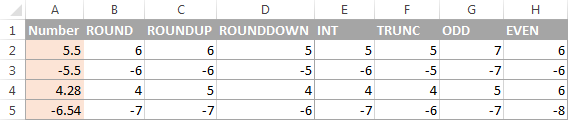### Round to nearest 0.5

Microsoft Excel provides 3 functions that let you round numbers to nearest half, more precisely to the nearest multiple of 0.5. Which one to use depends on your rounding criteria.

• To round a number down to nearest 0.5, use the FLOOR function, for example `=FLOOR(A2, 0.5)`.
• To round a number up to nearest 0.5, use the CEILING function, for example `=CEILING(A2, 0.5)`.
• To round a number up or down to nearest 0.5, use the MROUND function, e.g. `=MROUND(A2, 0.5)`. Whether MROUND rounds the number up or down depends on the remainder from dividing the number by multiple. If the remainder is equal to or greater than half the value of multiple, the number is rounded upward, otherwise downward.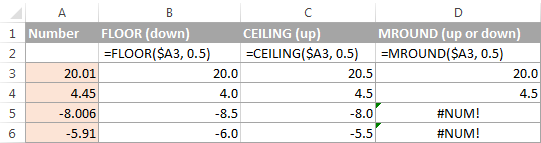As you see, the MROUND function can be used for rounding positive values only, when applied to negative numbers, it returns the #NUM error.

### Round to nearest 5 / 10 / 100 / 1000

Rounding to nearest five, ten, hundred or thousand is done in the same manner as rounding to 0.5 discussed in the previous example.

#### Round to nearest 5

Supposing that the number you want to round to closest 5 resides in cell A2, you can use on of the following formulas:

• To round a number down to nearest 5:
`=FLOOR(A2, 5)`
• To round a number up to nearest 5:
`=CEILING(A2, 5)`
• To round a number up or down to nearest 5:
`=MROUND(A2, 5)`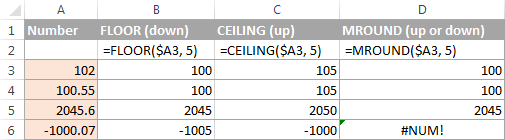#### Round to nearest 10

To round your numbers to nearest ten, supply 10 in the second argument of the rounding functions:

• To round a number down to nearest 10:
`=FLOOR(A2, 10)`
• To round a number up to nearest 10:
`=CEILING(A2, 10)`
• To round a number up or down to nearest 10:
`=MROUND(A2, 10)`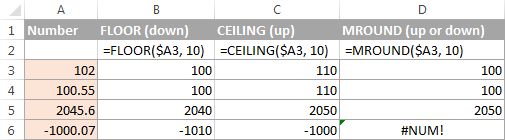#### Round to nearest 100

Rounding to a hundred is done in the same way, except that you enter 100 in the second argument:

• To round a number down to nearest 100:
`=FLOOR(A2, 100)`
• To round a number up to nearest 100:
`=CEILING(A2, 100)`
• To round a number up or down to nearest 100
`=MROUND(A2, 100)`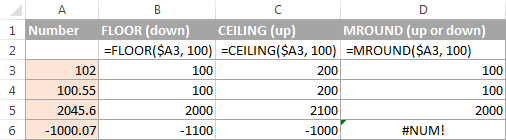#### Round to nearest 1000

To round a value in cell A2 to the nearest thousand, use of the following formulas:

• To round a number down to nearest 1000:
`=FLOOR(A2, 1000)`
• To round a number up to nearest 1000:
`=CEILING(A2, 1000)`
• To round a number up or down to nearest 1000
`=MROUND(A2, 1000)`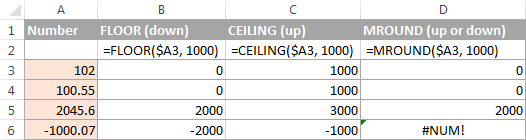The same techniques can be used for rounding numbers to other multiples. For example, you can round the prices to the nearest nickel (multiple of 0.05), lengths to the nearest inch (multiple of 1/12), or minutes to the nearest second (multiple of 1/60). Speaking of time, and do you know how to convert it to nearest hour or closest 5 or 10 minutes? If you don't, you will find the answers in the next section :)

### Rounding time in Excel

There may be many situations when you need to round time values. And again, you can use different rounding functions depending on your purpose.

#### Example 1. How to round time to nearest hour in Excel

With times located in column A, you can use one of the following functions to round time to nearest hour:

• To round time to closest hour (up or down) - MROUND or ROUND.

`=MROUND(A1,"1:00")`

`=MROUND(A1, TIME(1,0,0))`

`=ROUND(A1*24,0)/24`

• To round up time to nearest hour - ROUNDUP or CEILING.

`=CEILING(A1, "1:00")`

`=CEILING(A1, TIME(1,0,0))`

`=ROUNDUP(A1*24,0)/24`

• To round down time to nearest hour - ROUNDDOWN or FLOOR.

`=FLOOR(A1, "1:00")`

`=FLOOR(A1, TIME(1,0,0))`

`=ROUNDDOWN(A1*24,0)/24`

In the ROUND, ROUNDUP and ROUNDDOWN formulas, you multiply the time value by 24 (number of hours in a day) to convert a serial number representing the time to hours. Then you use one of the rounding functions to round the decimal value to an integer, and then divide it by 24 to change the returned value back to the time format.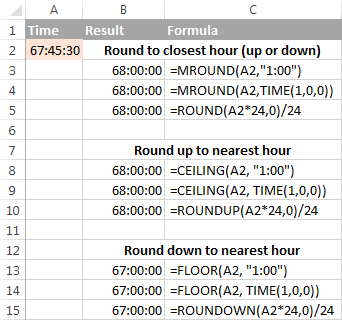If your timestamps include date values, then use the INT or TRUNC function to extract dates (in the internal Excel system, dates and times are stored as serial numbers, the integer part representing a date and fractional part representing time). And then, use the formulas described above but subtract the date value. For example:

`=MROUND(A1,"1:00") - INT(A1)`

`=MROUND(A1,"1:00") - TRUNC(A1)`

The following screenshot demonstrates other formulas: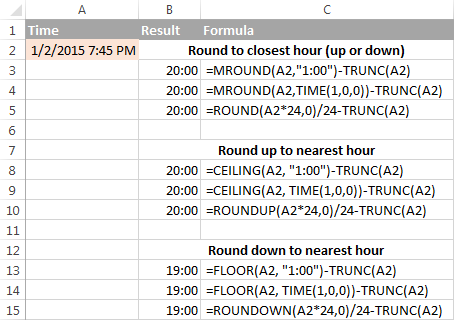Note. For the results to display correctly, remember to apply the Time format to your cells.

#### Example 2. Rounding time to nearest 5, 10, 15, etc. minutes

In case you want to round times in your Excel sheet to five or ten minutes, or to the closest quarter-hour, you can use the same rounding techniques as demonstrated above, but replace "1 hour" with the desired number of minutes in the formulas.

For example, to round the time in A1 to the closest 10 minutes, use one of the following functions:

• To round time to closest 10 minutes (up or down):`=MROUND(A1,"0:10")`

`=MROUND(A1, TIME(0,10,0))`

• To round up time to nearest 10 min:`=CEILING(A1, "0:10")`

`=CEILING(A1, TIME(0,10,0))`

• To round down time to nearest 10 min:`=FLOOR(A1, "0:10")`

`=FLOOR(A1, TIME(0,10,0))`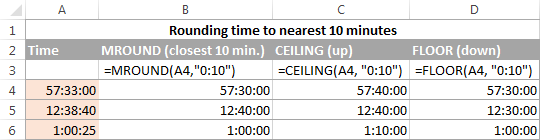If you know (or can calculate) what portion of a day is constituted by a certain number of minutes, then you won't have problems using the ROUND, ROUNDUP and ROUNDOWN functions as well.

For example, knowing that 15 minutes, is 1/96th of a day, you can use one of the following formulas to round the time in A1 to the nearest quarter-hour.

• To round time to closest 15 minutes (up or down): `=ROUND(A1*96,0)/96`
• To round up time to nearest 15 min: `=ROUNDUP(A1*96,0)/96`
• To round down time to nearest 15 min: `=ROUNDDOWN(A1*96,0)/96`

This is how you perform rounding in Excel. Hopefully, now you know how, among all those round functions, chose the one best suited for your needs.

### 166 responses to "How to round numbers in Excel - ROUND, ROUNDUP, ROUNDDOWN and other functions"

1.visu says:

thank you!! it helps so much

2.Jeric says:

Hi All,

I need you help on how to make this number 0.282186949 to become 28.21.

•Alexander Trifuntov (Ablebits.com Team) says:

Hello Jeric!
If I understand your task correctly, the following formula should work for you:

=ROUNDDOWN(A1*100,2)
or
=TRUNC(A1*100,2)

I hope this will help, otherwise please do not hesitate to contact me anytime.

3.Todd Bezold says:

Hello, I want to round a value either 1.5 or 1.4 to the nearest whole number 2.0. But if that same cell is 2.0 then the return cell would show 2.0.

•Alexander Trifuntov (Ablebits.com Team) says:

Hello Todd!
If I understand your task correctly, maybe the following formula should work for you:

=ROUNDUP(A1,0)

4.Oldie says:

Hi, great article but I still can't solve my problem, I have a set number to round up to e.g. Power Supplies come in 2,3,5,8,10,15 Amps, so if my load is 6.1 I need to choose 8A unit, or load is 10.8 chose the 15A unit

5.Naresh says:

excel formula in above 6 roundup 10 or below 5 roundown 0 if condition separate cell
mulitple purpose

•Alexander Trifuntov (Ablebits.com Team) says:

Hello Naresh!
The formula below will do the trick for you

=ROUND(C1,-1)

6.Paul-Simon MULDOWNEY says:

Hello, I would like to bypass the normal rounding function. I would like a number between 1.01 - 1.4 to round down and 1.41-1.99 to round up.

Is this possible?

•Alexander Trifuntov (Ablebits.com Team) says:

Hello Paul-Simon!
If I understand your task correctly, the following formula should work for you:

=IF(A20-TRUNC(A20) <= 0.4,ROUND(A20,0), ROUND(A20+0.1,0))

I hope this will help

7.Lucky says:

What will be the output of following1) =sum (5,6). 2)= power(5,3). 3)=round(14.67) - round off by one digit after decimal. 4) =max(78,89,48). 5) =LOWER ("CORONA")

•Alexander Trifuntov (Ablebits.com Team) says:

Hello!
What does sum (5,6) or LOWER (“CORONA”) have to do with the ROUND function? For me to be able to help you better, please specify which formula you mean and describe the problem in more detail. Thank you.

8.John says:

Hi,
I'm trying to write an equation in Excel that rounds using the following rules:
1. If the extra digit is less than 5, drop the digit.
2. If the extra digit is greater than 5, drop it and increase the previous digit by one.
3. If the extra digit is five, then increase the previous digit by one if it is odd; otherwise do not change the previous digit.

I feel like I'm close to a solution by using the IF(AND(Find line of commands but can't get it to come together properly. Could you please help?
Thanks so much,
John

9.Declan says:

Hi
I’m trying to round figures for my retail prices in one excel column
For example, simplest way for me to explain
Anything with a price between 17.01 and 17.50 rounds to 17.45
Anything with a price between 17.51 and 17.99 I want to round to 17.95
Any help I can get with this is much appreciated
Regards,
Declan

•Alexander Trifuntov (Ablebits.com Team) says:

Hello Declan!
If I understand your task correctly, the following formula should work for you:

=IF((K1-TRUNC(K1))<=0.5,TRUNC(K1)+0.45,TRUNC(K1)+0.95)

10.Lynn says:

I'm trying to round 161-169 to 165 and 160 still remain 160 in one cell.
Thanks to much.

•Alexander Trifuntov (Ablebits.com Team) says:

Hello Lynn!

=IF(A4-ROUNDDOWN(A4,-1)>0,ROUNDDOWN(A4,-1)+5,A4)

I hope this will help

11.Christine says:

What formula would I need to use to round up to the nearest \$5? Example \$63 round up to \$65.
THANKS!!

12.kiran SN says:

Hi , I am trying to write a formula for total hours in excel .
4(hrs).22(Min) + 4(hrs).46(Min) = 8(Hrs).68(Min) (Answer should be 9 hrs 8 minutes.)
7(hrs).30(Min) + 5(hrs).30(Mins)= 12(Hrs).60(Min) (Answer should be 13 hrs)

•Alexander Trifuntov (Ablebits.com Team) says:

Hello!
If you write 04:42:00 in cell A1, and write 04:46:00 in cell B1, then the formula =A1 + B1 gives 09:08:00. Explain in detail how you got your result and I will try to help you

13.HEATH says:

I have a weight in one column and in the other column I am wanting to multiply it by 65% AND then round it up to the nearest increment of 5 using one formula. Example: My weight is 125 and I want to know 65% of that weight which is 81.25. I would like to round it up to 85. Can i do all of this in one cell. I have this so far =R3*0.65 , but I don't know how to use another function in the same cell to use that function AND round up to the nearest 5. I would like all my weights to end in 5 or 0 ie ( 85,95,100 etc..) Can you help me?

14.Anoop says:

Hello Everyone,
If I wish to round off all decimal numbers upto 0.4999 to 0.5 and all decimal numbers greater than or equal to 0.501 to 0.9999 to 1. i.e. if a decimal number is 17.1 then it should be converted to 17.5 and if a decimal number is 29.65 then it should be converted to 30. These numbers should be rounded so that we arrive at the correct calculations.

•Alexander Trifuntov (Ablebits.com Team) says:

Hello!
I hope you have studied the recommendations in the above tutorial.
Use the CEILING function

=CEILING(A1,0.5)

15.Justin Murcutt says:

Hi,

Is there a way to round up to the nearest specific time?
ie. round 12/09/2020 20:30 to the nearest 6:00am time

•Alexander Trifuntov (Ablebits.com Team) says:

Hello!
If I understand your task correctly, the following formula should work for you:

=TRUNC(CEILING(B1,"06:00"))+0.25

I hope this will help

•Justin Murcutt says:

Hi,

Thanks for your reply. It does mostly do what i was asking. Is there a way for it to round upwards only? Some of the cells i am using it on is rounding some numbers backwards to "6:00" ie. 14/9/2020 11:40 is getting rounded to 14/9/2020 6:00 as opposed to 15/9/2020 6:00

•Alexander Trifuntov (Ablebits.com Team) says:

Hello!
I ask you to immediately specify all the conditions.

=IF((B11-INT(B11))>0.25,INT(B11+1)+0.25,INT(B11)+0.25)

Hope this is what you need.

•Justin Murcutt says:

Hi,

Thanks for your reply. Essentially what is needed is column A is a variety of different days and different times for each different day. The cell that will have the answer will need to round column A in the same row forward to 6:00am regardless of what the date is.
ie
Column A Column B
15/09/2020 17:30 16/09/2020 6:00
15/09/2020 21:43 16/09/2020 6:00
16/09/2020 3:26 16/09/2020 6:00
16/09/2020 8:15 17/09/2020 6:00
16/09/2020 13:26 17/09/2020 6:00
17/09/2020 5:00 17/09/2020 6:00
19/09/2020 20:45 20/09/2020 6:00

16.Hayden says:

Hello all,
Just wanted to know if anyone have solution to the currency rounding requirement below:

Cent of 0.01 to 0.04 = Round down to 0 (zero)
Cent of 0.05 = Remain as 0.05
Cent of 0.06 to 0.09 = round up as 0.10

By law in our country the currency need to be rounded in such way due to 1 cent coin is no longer a legal tender.

•Alexander Trifuntov (Ablebits.com Team) says:
17.matty says: